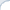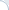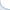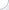## A small clarification on the LSH post

This is a clarification to the previous post. Note that I am not claiming that the LSH analysis is wrong! I am saying that apparently the analysis uses a simplifying assumption. And this assumption was swept under the carpet for many years (which is quite surprising). See also a discussion in the blog of Daniel Lemire.

Yet, such approaches are quite common in CS, statistics, physics, etc... As Daniel Lemire pointed out, an analysis of regular hash tables is essentially based on a very similar assumption: that a hash function distributes elements more or less randomly. I believe that in practice this is true only to a certain a degree (and this highly depends on a hash function). Yet, we still use the analysis. So, it is probably not a big deal.

I have recently come across another (actually well-known paper), where they talk at length about the assumptions being reasonable, but make a simple math error (or at least me and my co-author think so). So, it is infinitely better to rely (on perhaps silent) assumptions, but to get the math right, then to substantiate the assumptions and totally screw the math.﻿

## Does Locality Sensitive Hashing (LSH) analysis have a fatal flaw?

Preamble: Hongya Wang and colleagues have written a thought-provoking paper where they argue that performance analysis of a popular method: a Locality Sensitive Hashing (LSH) has a fatal flaw . Here is my take on this: even though their logic seems to be correct, I do not fully agree with their conclusions. In what follows, I consider this issue in more detail. See also my note here and a discussion in the blog of Daniel Lemire.

A locality-sensitive hashing, commonly known as LSH, is a popular method to compute locality-sensitive, distance-aware, hash values. More specifically, given two objects $x$ and $y$, the probability that their locality-sensitive hash values $h(x)$ and $h(y)$ are the same, depends on the distance between $x$ and $y$. The smaller is the distance, the more likely $x$ and $y$ collide with respect to the hash function $h()$. An event of getting $x$ and $y$ such that $h(x)=h(y)$ is called a collision.

This kind of distance sensitivity is a super-useful property, which fuels many probabilistic algorithms including approximate counting, clustering, and searching. In particular, with a good choice of a locality-sensitive function, we can build a hash table where close objects tend to be in the same bucket (with a sufficiently high probability). Thus, elements stored in the same bucket are good candidates for placing in the same cluster. In addition, given a query object $q$, potential nearest neighbors (again with some probability) can be found in the bucket corresponding to the hash value $h(q)$. If a collision probability for a single hash function is low, we need to build several hash tables over the same set. If we miss the nearest neighbor in one hash table, we still have a chance to find it another one. The more hash tables we build, the higher is a probability of success.2 Clearly, we need a family of locality-sensitive hash functions, but how do we get one?

In one specific, but important, case, we deal with bit (i.e., binary) vectors where dissimilarity is computed using the Hamming distance (the distance between bit vectors $x$ and $y$ is equal to the number of non-matching bits). In this setup, we can "create" binary hash functions by simply taking the value of an i-th bit. One can see that this is a projection to the 1-dimensional sub-space. The number of such functions $h^i()$ is equal to the length of a bit vector. Formally, the hash function number $i$ is defined as: $h^i(x) = x_i$.

This function is locality sensitive: The larger is the number of matching bits between vectors, the higher is the probability that they have equal i-th bits. What is the exact probability of a collision in this case? Typically, it is claimed to be $\frac{n - d}{n}$, where $n$ is the length of vectors and $d$ is the Hamming distance. For example, if vectors differ only in one bit, there is apparently $n$ ways to randomly select a projection dimension and, consequently, $n$ ways to select a hash function. Because, vectors differ only in a single bit number, only one choice of the hash function produces non-matching values. With respect to the remaining hash function, both vectors will collide. Thus, the collision probability is $\frac{n-1}{n}$.

Here is a subtle issue. As noted by Hongya Wang et al. , we do not randomly select a hash function for a specific pair of objects. Rather, we select hash functions randomly in advance and only afterwards we randomly select objects. Thus, we have a slightly different selection model than the one used in the previous paragraph! Using simple math, one can verify that if the set of objects comprises all possible bit vectors (and these bit vectors are selected randomly and uniformly), the two selection models are equivalent.

However, in general, these selection models are not equivalent. Consider a data set of bit vectors, such that their n-th bit is always set to one. For the first $n-1$ bits, all combinations are equally likely. Note that we essentially consider an (n-1)-dimensional sub-space with equi-probable elements. In this sub-space, two selection models are equivalent. Thus, a probability of a collision is $\frac{n - 1 - d}{n-1}$.

Note that this is true only for the first $n-1$ hash functions. Yet, for the function $h^n()$ the probability of a collision is different, namely it is one! Why? Because, we have a biased data set, where the value of the last (n-th) bit in each vector is always equal to one. Hence, a projection to the n-th dimension always results in a collision (for any pair of objects).

Hongya Wang et al.  go even further and carry out simulations. These simulations support their theoretical observations: The probabilities obtained via two different selection models are not always the same. So, it looks like the analysis of the LSH methods does rely on the simplifying assumption: The probability of a collision can be computed assuming that a hash function is randomly selected while the objects are fixed. Yet, I think that this simplification does not make a big difference, and it is the paper by Hongya Wang and colleagues that makes me think so.

First, even though collision ratios obtained via simulations (see Table 1 and Table 2 in the paper ) are sometimes quite different from predicted theoretical values, most of the time they are close to the theoretical predictions (again, based on the assumption that a hash function is selected randomly while objects are fixed). Second, as shown in Theorem 1, the average collision ratio rarely diverges from the theoretical value, if the number of objects and hash functions is large (which is mostly true in practice). Finally, I do not think that data sets where a significant fraction of the objects collide with respect to a given locality-sensitive hash function are likely. Such "mass" collisions might be a concern in some adversarial scenario (e.g., in the case of a DOS attack), but I would not expect this to happen under normal circumstances.

One nice property of the LSH is that we can tune parameters of the method to achieve a certain level of recall. Furthermore, these parameters can be selected based on the distribution of data . Thus, it would be possible to retrieve a true nearest neighbor in, e.g., about 50%, of all searches (in the shortest possible time). What happens if we miss the true answer? It is desirable that we still get something close. Yet, it turns out, that we often get results that are far from the nearest neighbor. This observation stems primarily from my own experiments , but there other papers where authors came to similar conclusions .

Thus, it may not be always possible to rely solely on the LSH in the nearest neighbor search. Streaming first story detection is one example, where authors use a hybrid method, in which an LSH-based search is a first step . If the LSH can find a tweet that is reasonably close to the query, the tweet topic is considered to be repetitive (i.e., it is not new). However, if we fail to find a previously created close-topic tweet using the LSH, we cannot be sure that the close-topic tweet does not exist. Why? The authors surmise that this may happen when the answer is not very close to the query. However, it might also be because the LSH tend to return a distant tweet even if a close-topic tweet exists in the index. To eliminate such potential false negatives, the authors use a small inverted file, which is built over a set of recent tweets.

To summarize, LSH is a great practical method based on reasonable theoretical assumptions, whose performance was verified empirically. It is possible to tune the parameters so that a certain recall level is achieved. Yet, there is seemingly no control in regard to how far are the objects in a result set from true nearest neighbors, when these true neighbors elude the LSH search algorithm.

1) This is a bit simplified description. In the real LSH, a hash function is often built using several elementary and binary hash functions, each of which is locality sensitive. In the case of real-valued vectors, one common approach to obtain binary functions is through a random projection.

## Efficient Intersections of compressed posting lists thanks to SIMD instructions

I co-authored a a paper on efficient intersection of compressed posting lists. I believe our team obtained good improvements over standard approaches using single instruction multiple data (SIMD) commands available on most modern processors. Here I want to briefly explain what was done and why it was hard to achieve these good improvements.

Parallelization of the algorithms is hard, but parallelization using a single CPU core is even harder. One of the first algorithms that exploited parallel capabilities of a single CPU were bit-parallel algorithms. For instance, if you carry out a bitwise AND between two 32-bit words, you essentially perform 32 boolean ANDs between 32 pairs of variables. Bitwise logical operations are, perhaps, the first SIMD instructions implemented by CPU designers. And thanks to Wikipedia, we know that the first bit-parallel algorithms were proposed more than 40 years ago.

More advanced CPUs may provide a rich set of SIMD instructions (far beyond bit-parallel logical operations). In particular, on x86 CPUs, you can efficiently carry out four additions over four pairs of single-precision floating numbers using a single SIMD instruction. Such instructions operate on small vectors and allow us to significantly improve performance of algorithms that process several streams of data in identical fashion, e.g., matrix multiplication algorithms. Somewhat surprisingly, SIMD operations can accelerate a lightweight compression of integers. By essentially splitting the data set into four parts, one can decompress billions of integers per second.

Unfortunately, CPUs provide only a limited set of control flow operations for vectorized data. In particular, x86-compatible CPUs have an instruction _mm_cmpeq_epi32 that checks element-wise equality of two integer vectors (each of which has four elements). However, this is not sufficient to parallelize an algorithm crucially relying on control flow instructions. Imagine that you compared four pairs of integers using _mm_cmpeq_epi32 and obtained the result in the form of a four-element bitmask (element i is 1 if and only if the the i-th pair have equal numbers). How do you extract matching integers? One clearly needs a gather-scatter instruction, but it is not supported by commodity x86 CPUs.

One devious workaround is to use a shuffle operation. To this end, one needs precomputed tables of shuffle masks. For example, if the comparison results can be interpreted as number 15, you retrieve the shuffle mask 15 from the shuffle-mask table and perform the shuffle operation. Accessing memory is not fast, even if data is in L1 cache. As an example, consider a problem of extracting integer values from sets represented as bitmaps. One well-known solution to this problem involves memoization. However, it is about five times slower than other approaches. If you do not believe, check the performance/code of the function bitscan4 in this sample code.

Now consider a C++ implementation of the classic textbook algorithm to carry out intersections of two sorted lists:

 while (first1!=last1 && first2!=last2)  {    if (*first1<*first2) ++first1;    else if (*first2<*first1) ++first2;    else {      *result = *first1;      ++result; ++first1; ++first2;    }  }

The algorithm has a complexity of $O(n+m)$ and is optimal in many cases ($m$, $n$ are list sizes) . However, it requires a lot of branches, which are often mispredicted. As a result, this algorithm is painfully slow: We estimate its performance to be about 300-400 million integers per second (the number of integers is computed as the sum of list sizes). Clearly, if you have an algorithm that can read a compressed posting list at the speed of 4 billion integers per second, it is not especially useful when the intersection algorithm is so tardy.

Schlegel et al proposed a neat way to vectorize this text-book algorithm. Their approach relies on all-against-all comparison SIMD instruction _mm_cmpestrm. Again, there is no gather-scatter instruction on x86 CPU. So, result extraction relies on the shuffle operation and requires reading of precomputed shuffle masks. Furthermore, even the counting version of this algorithm, which only evaluates the size of the intersection (without saving a result), is not especially efficient. We estimate that performance of this counting version is around 2 billion integers per second. It is much faster than the classic text-book algorithm, but is still an impediment, if the decompression algorithms has the speed of 4 billion integers per second.

More efficient (well-known) intersection algorithms exploit a differential in posting list size: Posting lists have different lengths and the differences are quite substantial. So, one can iterate over a shorter list and check if an element from the shorter list is present in the second list. For instance, the check can be done using a binary search (or a similar in spirit approach). Binary search relies on branching, thus, SIMD instructions should be useless here, right?

I would think so. Yet, Nathan Kurz figured out that it does not have to be the case here. He made a clever observation: When we are carrying out a binary search, eventually the search interval becomes so small that searching this interval sequentially becomes feasible. And such a sequential search can be accelerated using the instruction _mm_cmpeq_epi32 (that compares 4 pairs of integers at a time). This sequential scan can be carried out in a branchless fashion: Comparison results (one result is a bit mask) can be easily aggregated using the SIMD instruction _mm_or_si128. Furthermore, there is no extraction problem here. We iterate over the short list one integer at a time. Whenever, the integer is found in the second list, we memorize this common integer (which we already have in a scalar variable/register). Otherwise, we simply proceed to check the next one.

Nathan and Daniel (I wish I could claim credit here) turned this idea into an efficient intersection algorithm that beats the scalar checking version by a good margin. The performance can be further improved by storing some of the posting lists as bitmaps (the idea proposed by Culpepper and Moffat). As a result of these combined improvements, we achieve a sub-millisecond query-processing time for Gov2. For ClueWeb09 the query-processing time can be less than 2 milliseconds. Yet, I omit further details here and refer the reader to our joint paper.

In conclusion, our approach is based on a simple idea that was hard to come by. It was somewhat counter-intuitive that this simple method would work better than the approach due Schlegel et al, which employs the all-against-all comparison SIMD instruction. In fact, I think that the latter is quite useful when we intersect or merge lists of comparable sizes. However, this is probably not a typical scenario for a textual search engine.

## Main memory is similar to a hard drive

Today there was a first class of the large-scale machine learning course. If you run things at scale, you need methods with (near) linear run-time, i.e., $O(n)$ or $O(n \log(n))$. Not all big-O-es are created equal and constants do matter. Our instructor wanted to illustrate this statement. To this end, he used a well-known chart due to Peter Norvig and Jeff Dean, which highlights latencies of different memory types. As everybody remembers, computer memories are organized in a hierarchy. On top of this hierarchy is the fastest, but most expensive memory, whose size is small. At the bottom, there is a very cheap memory, e.g., a rotational or solid-state drive (SSD), which works slowly. Thus, reading $O(n)$ bytes from the hard drive is not the same as reading $O(n)$ bytes from the main memory: There is an orders-of-magnitude difference.

It is a rather well-known fact that the disk-based memory (even in the case of the SSD) is very slow. In fact, in many cases, it takes shorter time to read compressed data from the disk (and subsequently decompress it) rather than to read the uncompressed data. Another well-known fact is that the gap between the fastest volatile memory (CPU registers or L1 cache) and the slowest volatile memory is huge: A truly random read from main memory can cost you hundreds of CPU cycles. How many exactly?

The numbers in the Norvig-Dean's chart are a bit outdated, so I wrote a simple application to test memory speeds. I have a Core i7-4700MQ processor with the top frequency of 3.4Ghz. My computer has 16 GB of DDR3 memory. By running (use 12 GB of memory):

./test_mem_latency 12 0

I obtain that a random-access takes about 62 nanoseconds or approximately 200 CPU cycles. In contrast, it takes 0.3 nanoseconds to read one byte sequentially. These measurements are in line with tests made by other folks.

You can see that main memory is similar to the rotational hard drive in terms of performance: There is an orders-of-magnitude difference between the sequential and random-access reading speed. Furthermore, even though it is far less known, compressed data can also be read from memory faster than uncompressed data! For commodity servers, memory bandwidth rarely exceeds 10-20 GB/sec, and it is not hard to exhaust it if you have dozens of cores. A bit more surprising is the fact that you can read compressed data faster than uncompressed even if you employ only a single CPU core!

Main memory now resembles a hard drive. Thus, one benefits from using optimization techniques previously tailored to hard drives. One obvious optimization technique is caching. To this end, memory is divided into chunks. When we access a chunk that is not in the cache, a cache miss happens. This instructs a CPU to load this chunk into the cache.

There is also a division of memory into pages. Nowadays, programs access pages using logical addresses rather physical ones. Clearly, the CPU needs to translate logical addresses to physical addresses through a special translation table. Even if place such a table into a "fast" L1/L2 cache, a translation operation will be costly. This is why the CPU additionally employs a Tranlslation Lookaside Buffer. This buffer is a fast, but small cache. When the CPU is able to translate the page address using the TLB cache, we are lucky. Otherwise, an expensive TLB miss happens.

If we use huge pages, fewer translations (from logical to physical address) are required. We can provide efficient address translation for larger regions of memory, and, consequently, fewer TLB misses will happen. As was pointed out by Nathan Kurz, my memory benchmark can be easily modified to test the huge-page scenario. To do so, I allocate memory as follows (one needs Linux kernel 2.6.38 or later):

  if (useHugePage) { // As suggested by Nathan Kurz    mem = reinterpret_cast<char *>(mmap(NULL, MemSize, PROT_READ | PROT_WRITE,                                        MAP_PRIVATE| MAP_ANONYMOUS, -1, 0));    madvise(mem, MemSize, MADV_HUGEPAGE);  } else {    mem = new char[MemSize];  }

To enable the huge-page mode, one needs to run the benchmark with the following parameters (12 means that I will allocate 12GB)

./test_mem_latency 12 1

Now, I get a 1.5x improvement in the case of truly random access, and an amazing 5-fold improvement in the case of the semi-random (gapped) access.

Huge pages do come at a price. In particular, they may lead to a lower memory utilization (see also 4.3.2 of Ulrich Drepper paper). Yet, some memory inefficiencies can be tolerated (when speed is more important).

PS: I thank Nathan Kurz who proposed this optimization (including the code to allocate the memory) and memory speed-related references.

UPDATE: There was an inaccurate description of the paging mechanism. Thanks to Daniel Lemire who noticed this.

## Pages